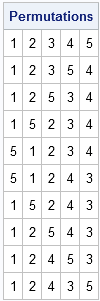In a previous post, I discussed how to generate random permutations of N elements. But what if you want to systematically iterate through a list of ALL permutations of N elements?

In the SAS DATA step you can use the ALLPERM subroutine in the SAS DATA step. For example, the following DATA step generates all permutations of five elements:

```%let n = 5; /** generate all permutations of n elements, in order **/ data perm&n (drop=i); array a{&n}; do i = 1 to &n; a[i]=i; end; /** initialize **/ do i = 1 to fact(&n); call allperm(i, of a[*]); output; end; run;```

This creates a SAS data set named PERM5, which has 5! = 120 rows.

Beginning with SAS 9.3, you can also use the ALLPERM function in SAS/IML software, as follows:

```proc iml; p5 = allperm(5); print (p5[1:10,])[label="Permutations"];```The complete set of 120 permutations is stored in the p5 matrix, but only the first few rows are displayed.

ShareDistinguished Researcher in Computational Statistics

Rick Wicklin, PhD, is a distinguished researcher in computational statistics at SAS and is a principal developer of SAS/IML software. His areas of expertise include computational statistics, simulation, statistical graphics, and modern methods in statistical data analysis. Rick is author of the books Statistical Programming with SAS/IML Software and Simulating Data with SAS.

1.Ankur biswas on

Dear Rick Wicklin Sir,
I'am pursuing Ph.D. in Agricultural Statistics from Indian Agricultural research Institute, New Delhi, India. I've read your blogs. Its encourages me to learn sas. Now-a-days i am trying to solve the pi i and pi ij for PPSwor scheme for any size of sample from a population of size N.
For that I need the probabilities of all possible samples (or permutations) and that has to be summed up. I can get that for small sample sizes. But for large sample sizes the permutations becomes so bigger.
With regards,

•I don't understand what you are asking, but here are two suggestions:
1) Read the PROC SURVEYSELECT documentation for PPS samples http://support.sas.com/documentation/cdl/en/statug/63033/HTML/default/viewer.htm#statug_surveyselect_sect007.htm.
2) If that doesn't answer your question, you might want to post your question to the following SAS Discussion Forum: http://communities.sas.com/community/sas_statistical_procedures

•Ankur biswas on

Actually I need to get all possible samples (permutations) of size n from a population of size N. For that all possible permutations of n symbols from N symbols are to be generated. But i don't need all permutations as a complete dataset. At the time of permutation generation, for each permutation i need a function of that permutation and that has to be summed up for all the permutations. Actually memory allocation problem arises at the time of permutation generation. The helpful programs shown in all of your blogs writes each permutation to a dataset.
With regards,

•I regret that I cannot offer personal assistance. For up to N<=18, you can look at the ALLPERM function in the DATA step. No computer can possibly generate all permutations for even modest values of N. Factorials grow really fast: 60! = 10^80 and even the world's fastest computer can only manage 10^16 computations per second. At that rate, it would require 10^64 seconds to compute all permutations, which is much longer than the age of the universe.

•Ankur biswas on

Thanks for your suggestion. I've already done it for N=25 using a combination of allcomb and allperm functions. But I need it for N=100. That's the problem. But if we don't store the whole permutation in a dataset and at the time of each permutation generation, if a function of that permutation is calculated and summed to a variables.

Is it possible? Then only my problem would be solved.
With regards,

•No, it's not possible to solve this with brute force. You'll have to use math.

2.Dear Rick Wicklin Sir,
Right now I am working on my Bachelor's thesis and encounter some problems with using IML.

I'm working on financial data, and trying to develop universe return and cumulative return of benchmark using IML/Macro.

```%let month = 5; %let startmonth = 5; %let endmonth = 5; %let startyear = 1999; %let endyear = 1999; %let numyears = %eval(&endyear-&startyear+1); %let nummonths = %eval(&endmonth-&startmonth+1);   %macro byyear; %do k = &startyear %to &endyear %by 1; data t&k; set toydata; where year=&k and month=&month; run; /*calculation*/ ods output avg_return&k = Areturn&k; proc iml; /* Set up array for the Chloe Matrix using &numyears for an array factor */ /* The allocaiton for j is nummonths, and the allocaiton for i is numyears*/ /* i is number of rown = years, and j is the portfolio, i.e., month */ use t&k; read all into a; return&k = a[,2]; weight&k = 1/ nRow(return&k);   nonMissing = loc(return&k ^=.); if ncol(nonMissing)=0 then mean = .; else do; return&k= return&k[nonMissing,]; avg_return&k= sum(return&k*weight&k); end; /* chloe(i,j) = avg_return&k */ print avg_return&k; quit; %end; %mend byyear; %byyear;```

I am able to get the value, 'avg_return&k.'After getting the avg_return&k, I want to put this value into another vector/array, to make it look like

```         Jan       Feb
1999 1.277      0.22
2000 0.988        ...
```

something looks like this. Would you be able to give me some recommendation?

Your book helped me a lot for my research. Thank you very much.
Sincerely, Chloe

•Chloe,
I suggest that your program will be simpler if you get rid of the macro %DO loop and use WHERE processing in SAS/IML instead. For an example, see my article "BY-group processing in SAS/IML." It will then be easy for you to use regular DO loops to loop over the YEAR and MONTH variables and fill in the elements of your array.